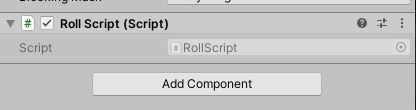# Public and SerializedField's not showing in inspector

I was creating a script for my dnd group so we could roll character stats, after writing my script they worked and I could see them in the inspector, but then the next time I opened Unity, my code wouldn’t run, it didn’t show any compiler errors or anything, just when I clicked play, the button that the code was attached to did nothing.

I soon realized that “[SerializeField]” and “public” do not show up in the inspector, and the objects I was passing into them had no reference.

I had to modify my code to find the objects by name instead, but I was wondering if anyone knew of a solution to the problem, as my next project will grab presets and import them through the inspector.

CODE:

``````using System;
using System.Collections.Generic;
using UnityEngine;
using UnityEngine.UI;
public class RollScript : MonoBehaviour
{
public static GameObject gameObjectPanel;
[SerializeField] static GameObject[] TextGameobject;
static Text[] texts;

void Start()
{
TextGameobject = new GameObject[] { GameObject.Find("Display00"), GameObject.Find("Display01"), GameObject.Find("Display10"), GameObject.Find("Display11"), GameObject.Find("Display20"), GameObject.Find("Display21"), GameObject.Find("Display3") };
texts = new Text[] { TextGameobject.GetComponent<Text>(), TextGameobject.GetComponent<Text>(), TextGameobject.GetComponent<Text>(), TextGameobject.GetComponent<Text>(), TextGameobject.GetComponent<Text>(), TextGameobject.GetComponent<Text>(), TextGameobject.GetComponent<Text>()
};
gameObjectPanel = GameObject.Find("Main_Panel");
}

public void Exit()
{
Application.Quit();
}

public void ActivateRoller()
{
//For testing purposes
if (Input.GetKey(KeyCode.Z))
{
RollGame(76, true);
}
else if (Input.GetKey(KeyCode.X))
{
RollGame(81, true);
}
else if (Input.GetKey(KeyCode.C))
{
RollGame(86, true);
}
else if (Input.GetKey(KeyCode.V))
{
RollGame(91, true);
}
//Stack overflow on following numbers
else if (Input.GetKey(KeyCode.B))
{
RollGame(96, true);
}
else if (Input.GetKey(KeyCode.N))
{
RollGame(101, true);
}
else
{
RollGame();
}
gameObjectPanel.SetActive(false);
}

static void RollGame(int minValue = 71, bool activated = false)
{
List<int> rollz = new List<int>();
rollz.Clear();
int wholeTotal = 0;
bool contains18 = false;
List<int> rollInt = new List<int>();
int lowInt;
if (!activated)
{
lowInt = 1;
}
else
{
lowInt = 2;
}
for (int i = 0; i < 6; i++)
{
rollInt.Clear();
for (int r = 0; r < 4; r++)
{
}
//foreach (var c in rollInt)
//{
//    Debug.Log("<color=red>"+c+"</color>");
//}
rollInt.Sort();
//foreach (var c in rollInt)
//{
//    Debug.Log("<color=green>" + c + "</color>");
//}
rollInt.RemoveAt(0);
//foreach (var c in rollInt)
//{
//    Debug.Log("<color=blue>" + c + "</color>");
//}
int total = 0;
foreach (var c in rollInt)
{
total += c;
}
if (total == 18)
{
contains18 = true;
}
wholeTotal += total;
}
if (wholeTotal < minValue)
{
//print("WHOLE TOTAL: " + wholeTotal);
RollGame(minValue, activated);
}
else
{
rollz.Sort();

Console.WriteLine("Contains 18: {0}", contains18);

//foreach (var c in rollz)
//{
//    print(c);
//}
for (int z = 0; z < 6; z++)
{
texts[z].text = rollz[z].ToString("D2");
}

texts.text = wholeTotal.ToString("D3");
}

}

public void backAction()
{
gameObjectPanel.SetActive(true);
}
}
``````

INSPECTOR:Hi!

Did you accidently made them static?

Because the reason you cannot see them in Inspector is because they are static - if you will remove static token you should be able see these variables in the Inspector.

For the information - you cannot see static variable in the Inspector because it’s the variable that shared between all instances of the class that are created, so it won’t make sense to be able set up it individually per each instance of script as it is acessed by its type, not instance.

You can find an workaround to expose static variables in the Inspector, but it’s more like about creating another variable that will be equal static variable and through another variable set up static variable.

Hope it helps.